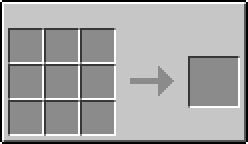# Mold (GregTech 6)

Mold

ModGregTech 6
TypeMachine
Technical details
Registry namegt.multitileentity:10XX
First appearance6.00.31

The Mold is a tile entity from GregTech 6 used to solidify molten material from the Smelting Crucible into various shapes.

## Recipe## Usage

Place the Mold adjacent to a Smelting Crucible and right-click on it. If there is material in the crucible, and the material is hot enough to be in liquid state, and there is enough material to fill the mold, the mold will be filled with the material and will begin cooling rapidly. The mold's contents will visibly change when the material cools down below its melting point, but the material may still be too hot to touch. Right-clicking the mold after the material solidifies will pick it up as an item, and will damage (and often kill) the player if it is too hot to touch. Wearing a complete Heat Protection Suit or Universal Hazard Suit, or holding a pair of Pincers, allows the player to safely take hot items from the mold.

### Automation

Items may be automatically extracted from below the mold using blocks such as Hoppers. The mold will only allow an item to be extracted after it has cooled to about room temperature. The mold may also be configured to draw and cast molten material from an adjacent Crucible using a Monkey Wrench. Right-click the wrench on any side of the mold to enable auto-casting from that direction. Right-click the wrench on the bottom of the mold to enable Redstone-controlled auto-casting (by default, auto-casting is always active).

Machines such as the Smelter can output molten material as standard Fluids. These fluids may be piped or inserted into any horizontal side of the mold, provided that enough fluid is being inserted to fill the mold. For example, the Mold may be placed next to the fluid output side of the machine and a hopper placed beneath it to automatically cast and cool the molten material.

Cryo energy, or CU, from a Thermoelectric Cooler can be used to cool down the mold and cast faster.

## Shapes

A mold has a 5x5 grid which can be chiseled out into a preferred shape. Only certain shapes are recognized and will function, but do not require a specific orientation or position on the mold. Note that there is currently no known way to undo chiseling a mold, so it is best for the user to careful while molding. An incorrectly chiseled mold will cast a number of nuggets equal to the number of chiseled squares. Any incorrectly chiseled mold may be chiseled further into a Plate mold, or the mold may be recycled in the Smelting Crucible or Shredder.

Here is a list of all the shapes that can be made in the Mold.

X = Chiseled O = Not chiseled.

### Ingot

 X X X X X X X X X X X X X X X

Consumes 1.000 units of material

### Chunk

 X X X X

Consumes 0.250 units of material

### Plate

 X X X X X X X X X X X X X X X X X X X X X X X X X

Consumes 1.000 units of material

### Tiny Plate

 X X X X X X X X X

Consumes 0.111 units of material

### Bolt

 X X

Consumes 0.125 units of material

### Item Casing

 X X X O X X X X O X X X X O X O O O O X X X X O O

Consumes 0.5 units of material

Raw items must be sharped with a Grindstone or Sanding Machine, or by crafting with a File.

 X X X X X O X X O

Consumes 3.000 units of material

 O X X X O X O O O X

Consumes 3.000 units of material

 X X X O X O O X O O X O O X O

Consumes 1.500 units of material

 X X X X X X X X X O X O O X O

Consumes 1.500 units of material

 X X X X X X X X X X X X O X O

Consumes 2.000 units of material

 X X X X X X X X X O X O

Consumes 1.000 units of material

 X X X X X X X X X X

Consumes 2.000 units of material

### Long Stick/Rod

 X O O O O O X O O O O O X O O O O O X O O O O O X

Consumes 1.000 units of material

### Stick/Rod

 X X X X X

Consumes 0.500 units of material

 X O O X X X X O O

Consumes 0.250 units of material

### Gear

 X O X O X O X X X O X X O X X O X X X O X O X O X

Consumes 4.000 units of material

### Small Gear

 O X O X O X X X X X O X O X O X X X X X O X O X O

Consumes 1.000 units of material

### Ring

 X X X X O X X X X

Consumes 0.250 units of material

 X X X X O X X X X O X X X X X X X X X O X X X X O

Consumes 4.000 units of material

 X X X O X X

Consumes 2.000 units of material

 X X X X X O X X X X

Consumes 3.000 units of material